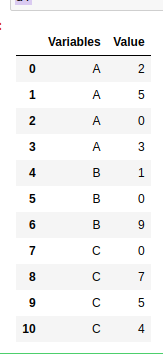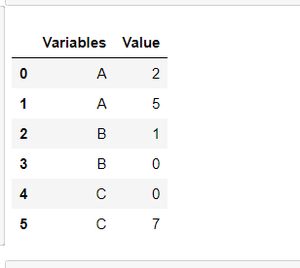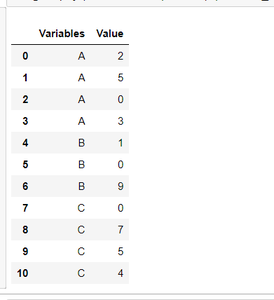Get topmost N records within each group of a Pandas DataFrame

• Last Updated : 28 Jul, 2020

Firstly, the pandas dataframe stores data in the form of a table. In some situations we need to retrieve data from dataframe according to some conditions. Such as if we want to get top N records of each group of the dataframe. Here we will use Groupby() function of pandas to group the columns. So we can do it as follows:

Firstly, we created a pandas dataframe:

Python3

 #importing pandas as pdimport pandas as pd  #creating dataframedf=pd.DataFrame({ 'Variables': ['A','A','A','A','B','B',                                'B','C','C','C','C'],                 'Value': [2,5,0,3,1,0,9,0,7,5,4]})df

Output:Now, we will get topmost N values of each group of the ‘Variables’ column. Here reset_index() is used to provide a new index according to the grouping of data. And head() is used to get topmost N values from the top.

Example 1: Suppose the value of N=2

Python3

 # setting value of N as 2N = 2  # using groupby to group acc. to# column 'Variable'df.groupby('Variables').head(N).reset_index(drop=True)

Output:Example 2: Now, suppose the value of N=4

Python3

 # setting value of N as 2N = 4  # using groupby to group acc. # to column 'Variable'df.groupby('Variables').head(N).reset_index(drop=True)

Output:My Personal Notes arrow_drop_up